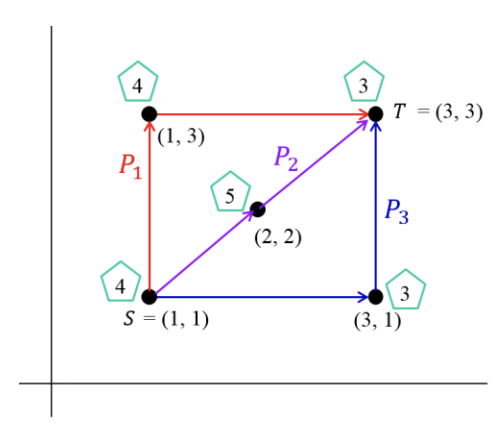시간 제한메모리 제한제출정답맞힌 사람정답 비율
2 초 512 MB94332531.250%

## 문제

An electric vehicle(EV) is an alternative fuel automobile which uses one or more electric motors for propulsion, in place of the internal combustion engine such as diesel engine or gasoline one. The EV stores electricity in an energy storage device, such as a battery, and the electricity powers the vehicle’s wheels via an electric motors. Due to its limited energy storage capacity, the EV must be regularly recharged by plugging into an electrical source.

There are n villages, referred to points on a plane. For arbitrary two villages, there is only one road between them. Thus, there are exactly n × (n − 1)/2 roads connecting villages each other. Let two villages A and B have coordinates (a, b) and (c, d), respectively. Then the length of the road between A and B is defined to be d(A, B) = |ac| + |bd|, which is called the distance between A and B. For convenience, it is assumed that the distance between A and B is equivalent to the amount of electricity needed for an EV to move between A and B. That is, while an EV consumes a unit electricity, say electricity 1, it can move a unit distance, say distance 1.

Each village has a charging station for an EV and the cost of charging may vary from village to village. Let c(A) be the cost per electricity 1 when an EV is charged in village A. By the above assumption, an EV with zero charge at village A must be charged at the cost of at least c(A)d(A, B) in A to arrive at village B.

Minsu should go from the village S to the village T by his EV. The EV is discharged, that is, with zero charge, at S before departure. Also the EV has a battery of maximum charge capacity W. That is, the EV cannot store the amount of electricity more than W. Thus if the EV is fully charged, then it can move as much as W. During the way from S to T, Minsu is allowed to make at most ∆ stops for recharging. Also the charging at the starting village S is considered as one of the stops for recharging. Then Minsu should find the cheapest way possible to move from S to T.For example, the above figure shows five villages in a plane with the costs of charging represented by the numbers in pentagons. Also, let W = 3 and ∆= 2. Three ways from S to TP1, P2, and P3, are shown as red, purple, and blue, respectively. In P1, the EV is charged by electricity 2 at S, and it is recharged by electricity 2 at (1, 3). So, totally the cost of 2 × 4 + 2 × 4 = 16 is incurred. For the case of P2, the EV is fully charged at S, and so the electricity 1 remains in the battery of EV when arriving at (2, 2). Then the EV is recharged at (2, 2) only by electricity 1 to reach T. So, totally the cost of 3 × 4 + 1 × 5 = 17 is incurred. Finally, in P3, the EV is charged by electricity 2 at S to move to (3, 1) and it is recharged by electricity 2 at (3, 1). So, totally the cost of 2 × 4 + 2 × 3 = 14 is incurred. The cost 14 is minimum and P3 is the cheapest way to move from S to T.

Given the coordinates of n villages including S and T, the charging cost at each village, the maximum charge capacity W of EV’s battery, and the arbitrary positive integer ∆, write a program to find the cheapest way to move from S to T with at most ∆ stops for recharging.

## 입력

Your program is to read from standard input. The input starts with an integer n (2 ≤ n ≤ 1,000), representing the number of villages. Each of the following n lines contains three integers, a, b, and c (0 ≤ a, b ≤ 106 and 1 ≤ c ≤ 104), where (a, b) is the coordinate of a village and c is the charging cost at the village. Here, in the n lines, the first line contains the coordinate of the starting village S and the second line contains the coordinate of the destination village T. Note that the coordinates of villages are all distinct. The next line contains an integer W (1 ≤ W ≤ 105) that represents the maximum charge capacity of EV’s battery. The last line contains a positive integer ∆ (1 ≤ ∆ ≤ 10), which indicates the maximum number of times for recharging while the EV travels from S to T. The charging at the starting village S is considered as one of the stops for recharging.

## 출력

Your program is to write to standard output. Print exactly one line. The line should contain the cost of the cheapest way for the EV to move from S to T with at most ∆ stops for recharging. If such a way does not exist, then the line should contain -1.

## 예제 입력 1

4
0 0 1
3 0 3
1 0 3
2 0 3
4
2


## 예제 출력 1

3


## 예제 입력 2

5
1 1 4
3 3 3
1 3 4
2 2 5
3 1 3
3
2


## 예제 출력 2

14


## 예제 입력 3

5
1 1 4
3 3 3
1 3 4
2 2 5
3 1 3
3
1


## 예제 출력 3

-1